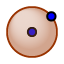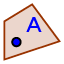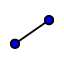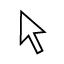# GeoGebra 3D Beginner Tutorial: Exercise 2

## NEW TOOLS WE WILL LEARN HOW TO USE:

Sphere with Center Through PointSphere with Center and RadiusMidpoint or CenterPoint on ObjectSegmentIn this exercise, we'll learn how to use these tools to explore the cross section of any two spheres that intersect in more than one point.

## DIRECTIONS:

1) Use the SPHERE WITH CENTER THROUGH POINTtool to create a sphere. Then select the MOVEarrow to deselect this sphere tool (i.e. turn this tool off). You should be able to drag point A (center of sphere) and B (which determines the sphere's radius). 2) Use the SPHERE with CENTER and RADIUStool to create a sphere with radius = 4. After doing so, select the MOVEarrow to deselect this sphere tool. 3) Move any one or both of these spheres around so that they intersect (overlap) each other. After doing so, right click on the gray plane. Uncheck "Show Plane" to hide this gray plane. 4) Use the INTERSECT 2 SURFACEStool. Select one sphere. Then select the other sphere. You should notice GeoGebra now plots the intersection of both of these 2 surfaces. Keep going: More directions appear below the applet.
5) Select the MOVE tool to deselect this tool you've just used in step (4). Move the spheres around now. Just be sure they still intersect each other. What does this intersection look like? 6) Now select the MIDPOINT OR CENTERtool. Select ONLY the intersection of these 2 surfaces (that you created in step 4). 7) Select the POINT ON OBJECTtool. Then select the intersection of both spheres (that you plotted in step (4)). Repeat this process again to put a second point on this intersection. 8) Use the SEGMENTtool to construct the segment connecting the point you created in steps (6) and any one of the 2 points you created in step (7). Then select the MOVE tool. Click on the segment to show the style bar. Click on the A icon. Deselect "Name" and select "value". Now move the endpoint of the segment on the intersection around. What do you notice?

## QUESTIONS:

What does your observations (from step 8) imply about the cross section (i.e. intersection) of both of these spheres? That is, if 2 spheres intersect in more than one point, how would you describe their intersection? Be sure to use another GeoGebra tool to help verify your assertion. Be sure to move both spheres around too. Is it possible for this intersection to ever change? Explain.

When you're done (or if you're unsure of something), feel free to check by watching the quick silent screencast below the applet.